# 114 Results

View
Selected filters:
• Calculus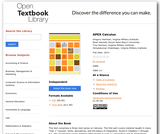Conditional Remix & Share Permitted
CC BY-NC
Rating

APEX Calculus is a calculus textbook written for traditional college/university calculus courses. It has the look and feel of the calculus book you likely use right now (Stewart, Thomas & Finney, etc.). The explanations of new concepts is clear, written for someone who does not yet know calculus. Each section ends with an exercise set with ample problems to practice & test skills (odd answers are in the back).

Subject:
Calculus
Material Type:
Textbook
Provider:
APEX: Affordable Print and Electronic TeXtbooks
Author:
Brian Heinold
Dimplekumar Chalishajar
Gregory Hartman
Troy Siemers
01/01/2014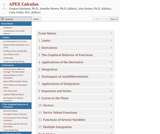Conditional Remix & Share Permitted
CC BY-NC
Rating

Textbook for Portland Community College Calculus sequence.

MTH 251: Includes limits, continuity, derivatives and some applications of derivatives.

MTH 252: Includes antiderivatives, the definite integral, topics of integration, improper integrals, and applications of differentiation and integration.

MTH 253: Includes infinite sequences and series (including Taylor series), vectors, and geometry of space.

MTH 254: Includes multivariate and vector-valued functions from a graphical, numerical, and symbolic perspective. Applies integration and differentiation of both types of functions to solve real world problems.

Subject:
Calculus
Material Type:
Textbook
Author:
Alex Jordan
Carly Vollet
Gregory Hartman
Jennifer Bowen
06/24/2019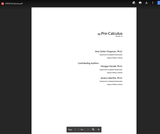Conditional Remix & Share Permitted
CC BY-NC
Rating

This text was written as a prequel to the APEXCalculus series, a three–volume series on Calculus. This text is not intended to fully prepare students with all of the mathematical knowledge they need to tackle Calculus, rather it is designed to review mathematical concepts that are often stumbling blocks in the Calculus sequence. It starts basic and builds to more complex topics. This text is written so that each section and topic largely stands on its own, making it a good resource for students in Calculus who are struggling with the supporting mathemathics found in Calculus courses. The topics were chosen based on experience; several instructors in the Applied Mathemathics Department at the Virginia Military Institute (VMI) compiled a list of topics that Calculus students commonly struggle with, giving the focus of this text. This allows for a more focused approach; at first glance one of the obvious differences from a standard Pre-Calculus text is its size.

Subject:
Calculus
Material Type:
Textbook
Author:
Amy Givler Chapman
Jessica Libertini
Meagan Herald
06/28/2019Conditional Remix & Share Permitted
CC BY-NC-SA
Rating

Active Calculus is different from most existing calculus texts in at least the following ways: the text is free for download by students and instructors in .pdf format; in the electronic format, graphics are in full color and there are live html links to java applets; the text is open source, and interested instructors can gain access to the original source files upon request; the style of the text requires students to be active learners — there are very few worked examples in the text, with there instead being 3-4 activities per section that engage students in connecting ideas, solving problems, and developing understanding of key calculus concepts; each section begins with motivating questions, a brief introduction, and a preview activity, all of which are designed to be read and completed prior to class; the exercises are few in number and challenging in nature.

Subject:
Mathematics
Calculus
Material Type:
Textbook
Provider:
Grand Valley State University
Author:
David Austin
Matt Boelkins
Steve Schlicker
02/19/2015Conditional Remix & Share Permitted
CC BY-NC-SA
Rating

Active Calculus Multivariable is the continuation of Active Calculus to multivariable functions. The Active Calculus texts are different from most existing calculus texts in at least the following ways: the texts are free for download by students and instructors in .pdf format; in the electronic format, graphics are in full color and there are live html links to java applets; the texts are open source, and interested instructors can gain access to the original source files upon request; the style of the texts requires students to be active learners — there are very few worked examples in the texts, with there instead being 3-4 activities per section that engage students in connecting ideas, solving problems, and developing understanding of key calculus concepts; each section begins with motivating questions, a brief introduction, and a preview activity, all of which are designed to be read and completed prior to class; the exercises are few in number and challenging in nature.

Subject:
Calculus
Material Type:
Textbook
Provider:
Grand Valley State University
Author:
David Austin
Matthew Boelkins
Steven Schlicker
07/01/2018Conditional Remix & Share Permitted
CC BY-NC-SA
Rating

Some of the topics that this book addresses are: Vector spaces; finite-dimensional vector spaces; differential calculus; compactness and completeness; scalar product space; differential equations; multilenear functionals; integration; differentiable manifolds; integral calculus on manifolds; exterior calculus.

Note: this is a 57 MB PDF Document.

Subject:
Calculus
Material Type:
Textbook
Provider:
Harvard University
Provider Set:
Individual Authors
Author:
Sternberg Shlomo and Lynn Loomis
02/16/2011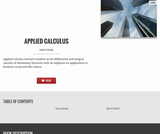Rating

Applied Calculus instructs students in the differential and integral calculus of elementary functions with an emphasis on applications to business, social and life science. Different from a traditional calculus course for engineering, science and math majors, this course does not use trigonometry, nor does it focus on mathematical proofs as an instructional method.

Subject:
Calculus
Material Type:
Full Course
Textbook
Provider:
Lumen Learning
Provider Set:
Candela Courseware
Author:
Dale Hoffman
David Lippman
Shana Calaway
03/31/2016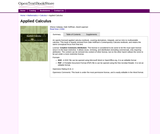Unrestricted Use
CC BY
Rating

An openly licensed applied calculus textbook, covering derivatives, integrals, and an intro to multivariable calculus. This book is heavily remixed from Dale Hoffman's Contemporary Calculus textbook, and retains the same conceptual focus from that text.

Subject:
Calculus
Material Type:
Textbook
Author:
Dale Hoffman
David Lippman
Shana Calaway
08/14/2020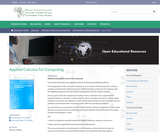Unrestricted Use
CC BY
Rating

Welcome to applied calculus for computing. Calculation intended to be a general method of solving quantifiable problems. In the application of the calculation method, or as it is known the”infinitesimal” method, a problem is”divided into infinitesimal parts”(differentiation), analysed in its relations with the neighbouring parts and then”added”(integration) until the solution method. The two parts of this the analysis and synthesis form a model for more sophisticated methods based on calculation, used in applied science concepts you learn in calculus allow statistical , physicists and engineers create mathematical models of real situations and real problems and simulate their resolutions under different operating conditions.

Subject:
Calculus
Material Type:
Module
Provider:
African Virtual University
Provider Set:
OER@AVU
Author:
Willian Munyiri
02/10/2018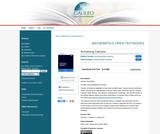Conditional Remix & Share Permitted
CC BY-NC-SA
Rating

The text is mostly an adaptation of two other excellent open- source calculus textbooks: Active Calculus by Dr. Matt Boelkins of Grand Valley State University and Drs. Gregory Hartman, Brian Heinold, Troy Siemers, Dimplekumar Chalishajar, and Jennifer Bowen of the Virginia Military Institute and Mount Saint Mary's University. Both of these texts can be found at http://aimath.org/textbooks/approved-textbooks/.
The authors of this text have combined sections, examples, and exercises from the above two texts along with some of their own content to generate this text. The impetus for the creation of this text was to adopt an open-source textbook for Calculus while maintaining the typical schedule and content of the calculus sequence at our home institution.

Subject:
Calculus
Material Type:
Textbook
Provider:
University System of Georgia
Provider Set:
Galileo Open Learning Materials
Author:
Jared Schlieper,
Michael Tiemeyer
03/20/2015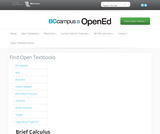Unrestricted Use
CC BY
Rating

This short text is designed more for self-study or review than for classroom use; full solutions are given for nearly all the end-of-chapter problems. For a more traditional text designed for classroom use, see Fundamentals of Calculus (http://www.lightandmatter.com/fund/). The focus is mainly on integration and differentiation of functions of a single variable, although iterated integrals are discussed. Infinitesimals are used when appropriate, and are treated more rigorously than in old books like Thompson's Calculus Made Easy, but in less detail than in Keisler's Elementary Calculus: An Approach Using Infinitesimals. Numerical examples are given using the open-source computer algebra system Yacas, and Yacas is also used sometimes to cut down on the drudgery of symbolic techniques such as partial fractions. Proofs are given for all important results, but are often relegated to the back of the book, and the emphasis is on teaching the techniques of calculus rather than on abstract results.

Subject:
Mathematics
Calculus
Material Type:
Textbook
Provider:
BCcampus
Provider Set:
BCcampus Open Textbooks
Author:
Benjamin Crowell
10/28/2014Rating

This course provides an introduction to applied concepts in Calculus that are relevant to the managerial, life, and social sciences. Students should have a firm grasp of the concept of functions to succeed in this course. Topics covered include derivatives of basic functions and how they can be used to optimize quantities such as profit and revenues, as well as integrals of basic functions and how they can be used to describe the total change in a quantity over time.

Subject:
Calculus
Material Type:
Full Course
Textbook
Provider:
Lumen Learning
Provider Set:
Candela Courseware
03/31/2016Unrestricted Use
CC BY
Rating

MATH&148 is a calculus course for business students. It is designed for students who want a brief course in calculus. Topics include differential and integral calculus of elementary functions. Problems emphasize business and social science applications. Translating words into mathematics and solving word problems are emphasized over algebra. Applications are mainly business oriented (e.g. cost, revenue, and profit). Mathematical theory and complex algebraic manipulations are not mainstays of this course, which is designed to be less rigorous than the calculus sequence for scientists and engineers. Topics are presented according to the rule of four: geometrically, numerically, analytically, and verbally. That is, symbolic manipulation must be balanced with graphical interpretation, numerical examples, and writing. Trigonometry is not part of the course.

Subject:
Calculus
Material Type:
Assessment
Full Course
Syllabus
Textbook
Provider:
Washington State Board for Community & Technical Colleges
Provider Set:
Open Course Library
05/03/2013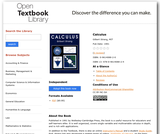Conditional Remix & Share Permitted
CC BY-NC-SA
Rating

Published in 1991 by Wellesley-Cambridge Press, the book is a useful resource for educators and self-learners alike. It is well organized, covers single variable and multivariable calculus in depth, and is rich with applications.

In addition to the Textbook, there is also an online Instructor's Manual and a student Study Guide. Prof. Strang has also developed a related series of videos, Highlights of Calculus, on the basic ideas of calculus.

Subject:
Mathematics
Calculus
Material Type:
Full Course
Provider:
MIT
Author:
Gilbert Strang
01/01/1991Unrestricted Use
CC BY
Rating

This is a four unit module. The first two units cover the basic concepts of the differential and integral calculus of functions of a single variable. The third unit is devoted to sequences of real numbers and infinite series of both real numbers and of some special functions. The fourth unit is on the differential and integral calculus of functions of several variables.

Subject:
Calculus
Material Type:
Module
Provider:
African Virtual University
Provider Set:
OER@AVU
Author:
Ralph W.P. Masenge
03/14/2018Rating

Calculus is the mathematics of CHANGE and almost everything in our world is changing. In this course, you will investigate limits and how they are used to calculate rate of change at a point, define the continuity of a function and how they are used to define derivatives. Definite and indefinite integrals and their applications are covered, including improper integrals. Late in the course, you will find Calculus with parametric equations and polar coordinates, sequences and series, and vectors.

Subject:
Calculus
Material Type:
Full Course
Textbook
Provider:
Lumen Learning
Provider Set:
Candela Courseware
Author:
Dale Hoffman
David Lippman
Jeff Eldridge
Melonie Rasmussen
03/31/2016Conditional Remix & Share Permitted
CC BY-NC-SA
Rating

This series of videos focusing on calculus covers sample questions from the A.P, Calculus AB and AC exams (both multiple choice and free answer).

Subject:
Calculus
Material Type:
Lecture
Provider:
Provider Set:
05/28/2013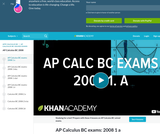Conditional Remix & Share Permitted
CC BY-NC-SA
Rating

The Calculus BC AP exam is a super set of the AB exam. It covers everything in AB as well as some of the more advanced topics in integration, sequences and function approximation. This tutorial is great practice for anyone looking to test their calculus mettle!

Subject:
Calculus
Material Type:
Lecture
Provider:
Provider Set:
Author:
Salman Khan
05/30/2013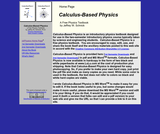Conditional Remix & Share Permitted
CC BY-SA
Rating

Calculus-Based Physics is an introductory physics textbook designed for use in the two-semester introductory physics course typically taken by science and engineering students.

Subject:
Calculus
Physics
Material Type:
Textbook
Provider:
Saint Anselm College
Author:
Jeffrey Schnick
02/16/2011Conditional Remix & Share Permitted
CC BY-NC-SA
Rating

This series of videos focusing on calculus covers minima, maxima, and critical points, rates of change, optimization, rates of change, L'Hopital's Rule, mean value theorem.

Subject:
Calculus
Material Type:
Lecture
Provider:
Provider Set:
05/28/2013Conditional Remix & Share Permitted
CC BY-NC-SA
Rating

A single definite integral can be used to find the area under a curve. with double integrals, we can start thinking about the volume under a surface!

Subject:
Calculus
Material Type:
Lecture
Provider:
Provider Set:
Author:
Salman Khan
05/30/2013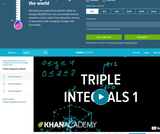Conditional Remix & Share Permitted
CC BY-NC-SA
Rating

This is about as many integrals we can use before our brains explode. Now we can sum variable quantities in three-dimensions (what is the mass of a 3-D wacky object that has variable mass)!

Subject:
Calculus
Material Type:
Lecture
Provider:
Provider Set:
Author:
Salman Khan
05/30/2013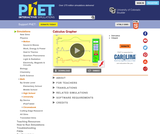Unrestricted Use
CC BY
Rating

Draw a graph of any function and see graphs of its derivative and integral. Don't forget to use the magnify/demagnify controls on the y-axis to adjust the scale.

Subject:
Calculus
Material Type:
Simulation
Provider:
Provider Set:
PhET Interactive Simulations
Author:
Michael Dubson
Trish Loeblein
08/31/2009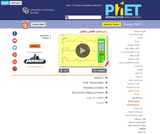Unrestricted Use
CC BY
Rating

Draw a graph of any function and see graphs of its derivative and integral. Don't forget to use the magnify/demagnify controls on the y-axis to adjust the scale.

Subject:
Calculus
Material Type:
Simulation
Provider:
Provider Set:
PhET Interactive Simulations
Author:
Michael Dubson
Patricia Loblein
02/02/2013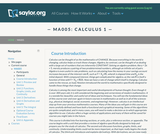Unrestricted Use
CC BY
Rating

This course begins with a review of algebra specifically designed to help and prepare the student for the study of calculus, and continues with discussion of functions, graphs, limits, continuity, and derivatives. The appendix provides a large collection of reference facts, geometry, and trigonometry that will assist in solving calculus problems long after the course is over. Upon successful completion of this course, the student will be able to: calculate or estimate limits of functions given by formulas, graphs, or tables by using properties of limits and LĺÎĺ_ĺĚĺ_hopitalĺÎĺ_ĺĚĺ_s Rule; state whether a function given by a graph or formula is continuous or differentiable at a given point or on a given interval and justify the answer; calculate average and instantaneous rates of change in context, and state the meaning and units of the derivative for functions given graphically; calculate derivatives of polynomial, rational, common transcendental functions, and implicitly defined functions; apply the ideas and techniques of derivatives to solve maximum and minimum problems and related rate problems, and calculate slopes and rates for function given as parametric equations; find extreme values of modeling functions given by formulas or graphs; predict, construct, and interpret the shapes of graphs; solve equations using NewtonĺÎĺ_ĺĚĺ_s Method; find linear approximations to functions using differentials; festate in words the meanings of the solutions to applied problems, attaching the appropriate units to an answer; state which parts of a mathematical statement are assumptions, such as hypotheses, and which parts are conclusions. This free course may be completed online at any time. It has been developed through a partnership with the Washington State Board for Community and Technical Colleges; the Saylor Foundation has modified some WSBCTC materials. (Mathematics 005)

Subject:
Calculus
Functions
Material Type:
Assessment
Full Course
Syllabus
Textbook
Provider:
The Saylor Foundation
04/16/2012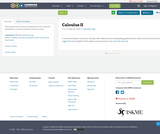Conditional Remix & Share Permitted
CC BY-NC-SA
Rating

This site provides lectures and guided lecture notes for students for a second-semester calculus course.

Subject:
Calculus
Material Type:
Lecture
Lecture Notes
Author:
Kamuela Yong
05/23/2019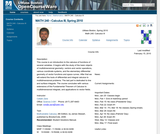Conditional Remix & Share Permitted
CC BY-NC-SA
Rating

This course is an introduction to the calculus of functions of several variables. It begins with studying the basic objects of multidimensional geometry: vectors and vector operations, lines, planes, cylinders, quadric surfaces, and various coordinate systems. It continues with the elementary differential geometry of vector functions and space curves. After this, it extends the basic tools of differential calculus - limits, continuity, derivatives, linearization, and optimization - to multidimensional problems. The course will conclude with a study of integration in higher dimensions, culminating in a multidimensional version of the substitution rule.

Subject:
Calculus
Geometry
Material Type:
Full Course
Homework/Assignment
Syllabus
Provider:
UMass Boston
Provider Set:
UMass Boston OpenCourseWare
Author:
Catalin Zara
Ph.D.
02/16/2011Unrestricted Use
CC BY
Rating

This contemporary calculus course is the third in a three-part sequence. In this course students continue to explore the concepts, applications, and techniques of Calculus - the mathematics of change. Calculus has wide-spread application in science, economics and engineering, and is a foundation college course for further work in these areas. This is a required class for most science and mathematics majors.Login: guest_oclPassword: ocl

Subject:
Calculus
Material Type:
Full Course
Homework/Assignment
Lecture Notes
Lesson Plan
Syllabus
Provider:
Washington State Board for Community & Technical Colleges
Provider Set:
Open Course Library
10/31/2011Unrestricted Use
CC BY
Rating

This contemporary calculus course is the second in a three-part sequence. In this course students continue to explore the concepts, applications, and techniques of Calculus - the mathematics of change. Calculus has wide-spread application in science, economics and engineering, and is a foundation college course for further work in these areas. This is a required class for most science and mathematics majors.Login: guest_oclPassword: ocl

Subject:
Calculus
Material Type:
Full Course
Homework/Assignment
Lecture Notes
Lesson Plan
Syllabus
Provider:
Washington State Board for Community & Technical Colleges
Provider Set:
Open Course Library
10/31/2011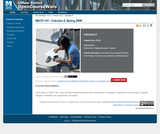Conditional Remix & Share Permitted
CC BY-NC-SA
Rating

Topics in this course include transcendental functions, techniques of integration, applications of the integral, improper integrals, l'Hospital's rule, sequences, and series.

Subject:
Calculus
Material Type:
Full Course
Homework/Assignment
Syllabus
Provider:
UMass Boston
Provider Set:
UMass Boston OpenCourseWare
Author:
Catalin Zara
Ph.D.
02/16/2011Unrestricted Use
CC BY
Rating

This course is an introduction to contemporary calculus and is the first of a three-part sequence. In this course students explore the concepts, applications, and techniques of Calculus - the mathematics of change. Calculus has wide-spread application in science, economics and engineering, and is a foundation college course for further work in these areas. This is a required class for most science and mathematics majors.Login: guest_oclPassword: ocl

Subject:
Calculus
Functions
Material Type:
Full Course
Homework/Assignment
Lecture Notes
Lesson Plan
Syllabus
Provider:
Washington State Board for Community & Technical Colleges
Provider Set:
Open Course Library
10/31/2011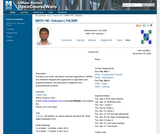Conditional Remix & Share Permitted
CC BY-NC-SA
Rating

This course is an introduction to differential and integral calculus. It begins with a short review of basic concepts surrounding the notion of a function. Then it introduces the important concept of the limit of a function, and use it to study continuity and the tangent problem. The solution to the tangent problem leads to the study of derivatives and their applications. Then it considers the area problem and its solution, the definite integral. The course concludes with the calculus of elementary transcendental functions.

Subject:
Calculus
Material Type:
Full Course
Homework/Assignment
Syllabus
Provider:
UMass Boston
Provider Set:
UMass Boston OpenCourseWare
Author:
Catalin Zara
02/16/2011Conditional Remix & Share Permitted
CC BY-NC-SA
Rating

This series of videos focusing on calculus covers indefinite integral as anti-derivative, definite integral as area under a curve, integration by parts, u-substitution, trig substitution.

Subject:
Calculus
Material Type:
Lecture
Provider:
Provider Set:
05/28/2013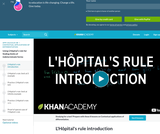Conditional Remix & Share Permitted
CC BY-NC-SA
Rating

This 9-minute video lesson provides an introduction to L'Hopital's Rule. [Calculus playlist: Lesson 36 of 156]

Subject:
Calculus
Material Type:
Lecture
Provider:
Provider Set:
Author:
Salman Khan
02/20/2011Conditional Remix & Share Permitted
CC BY-NC-SA
Rating

This series of videos focusing on calculus covers limit introduction, squeeze theorem, and epsilon-delta definition of limits.

Subject:
Calculus
Material Type:
Lecture
Provider:
Provider Set:
05/28/2013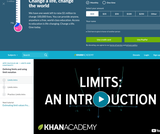Conditional Remix & Share Permitted
CC BY-NC-SA
Rating

Limits are the core tool that we build upon for calculus. Many times, a function can be undefined at a point, but we can think about what the function "approaches" as it gets closer and closer to that point (this is the "limit"). Other times, the function may be defined at a point, but it may approach a different limit. There are many, many times where the function value is the same as the limit at a point. Either way, this is a powerful tool as we start thinking about slope of a tangent line to a curve.

Subject:
Calculus
Material Type:
Lecture
Provider:
Provider Set:
05/30/2013Conditional Remix & Share Permitted
CC BY-NC-SA
Rating

This tutorial covers much of the same material as the "Limits" tutorial, but does it with Sal's original "old school" videos. The sound, resolution or handwriting isn't as good, but some people find them more charming.

Subject:
Calculus
Material Type:
Lecture
Provider:
Provider Set:
05/30/2013Conditional Remix & Share Permitted
CC BY-NC-SA
Rating

This series of videos focusing on calculus covers line integral of scalar and vector-valued functions, Green's Theorem and 2-D Divergence Teorem.

Subject:
Calculus
Material Type:
Lecture
Provider:
Provider Set:
05/28/2013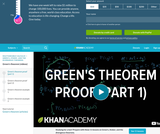Conditional Remix & Share Permitted
CC BY-NC-SA
Rating

It is sometimes easier to take a double integral (a particular double integral as we'll see) over a region and sometimes easier to take a line integral around the boundary. Green's theorem draws the connection between the two so we can go back and forth. This tutorial proves Green's theorem and then gives a few examples of using it. If you can take line integrals through vector fields, you're ready for Mr. Green.

Subject:
Calculus
Material Type:
Lecture
Provider: